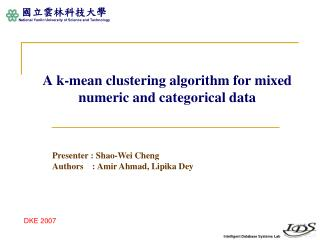Download PresentationA k-mean clustering algorithm for mixed numeric and categorical data

A k-mean clustering algorithm for mixed numeric and categorical data - PowerPoint PPT Presentation

A k-mean clustering algorithm for mixed numeric and categorical data. Presenter : Shao -Wei Cheng Authors : Amir Ahmad, Lipika Dey. DKE 2007. Outline. Motivation Objective Methodology Experiments Conclusion Comments. Motivation.I am the owner, or an agent authorized to act on behalf of the owner, of the copyrighted work described.
Download PresentationA k-mean clustering algorithm for mixed numeric and categorical data

Download Policy: Content on the Website is provided to you AS IS for your information and personal use and may not be sold / licensed / shared on other websites without getting consent from its author.While downloading, if for some reason you are not able to download a presentation, the publisher may have deleted the file from their server.

- - - - - - - - - - - - - - - - - - - - - - - - - - E N D - - - - - - - - - - - - - - - - - - - - - - - - - -
Presentation Transcript
1. A k-mean clustering algorithm for mixed numeric and categorical data Presenter : Shao-Wei Cheng Authors : Amir Ahmad, Lipika Dey DKE 2007

2. Outline • Motivation • Objective • Methodology • Experiments • Conclusion • Comments

3. Motivation • The traditional k-mean algorithm is limited to numeric data. • The Huang’s cost algorithm tried to cluster mixed numeric and categorical data • The cluster center is represented by the mode of the cluster. • Use the binary distance between two categorical attribute values. • The significance(weight) of numeric attribute is taken to be 1, and γjis a user-defined parameter. 3

4. Objectives • This paper attempts to alleviate the short-comings of Huang’s cost algorithm. • Propose a new representation for the cluster center. • Computing distance between two categorical values by the overall distribution of categorical attribute. • The parameter is defined by the contribution of a categorical attribute. 4

5. Methodology • Cost function • The Huang’s cost algorithm • The proposed cost algorithm The distance between De Niroand Stewart is ?

6. Methodology

7. Methodology • Significance of numeric attribute • The numeric attributes need to be discretized. • equal width discretization

8. Methodology • Algorithm • Initialization. • Computing the cluster centers. • Assign the data element to the cluster whose center is closest to it • Repeat 2 and 3, until clusters do not change or for a fixed number of iterations. 8

9. Experiments • Evaluation method • Data sets • Iris – all numeric attributes • Vote – all categorical attributes • Heart disease data – mixed data set • Australian credit data – mixed data set 9

10. Conclusion • This paper introduced a new distance measure for categorical attribute values and proposed a modified k-mean algorithm for clustering mixed data sets. • The results obtained with this algorithm over a number of real-world data sets are highly encouraging. • Future work • Other methods for discretizing numeric valued attributes. • Other implementations of k-mean algorithm. 11

11. Comments • Advantage • The view of overall attributes is good. • Drawback • … • Application • Mixed data sets clustering.